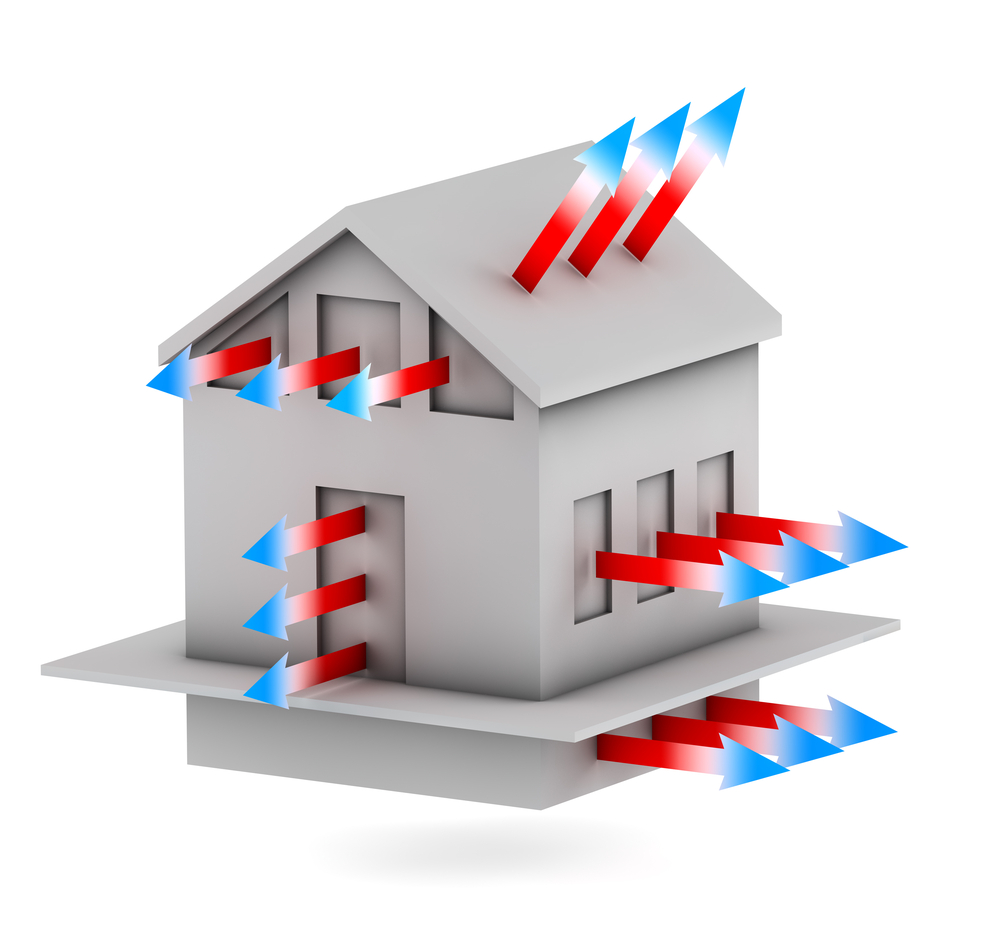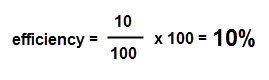# Energy Efficiency

In this worksheet, students will consider how energy can be used more efficiently and how that impacts on the cost of energy.Key stage:  KS 3

Curriculum topic:   Physics: Energy

Curriculum subtopic:   Energy Changes and Transfers

Difficulty level:### QUESTION 1 of 10

Household energy bills are increasing year on year. Due to this large increase in energy prices, more and more households are looking at how they can prevent energy loss from their home and reduce their energy bills. Most of the energy wasted in a home is due to heat loss.There are several ways to reduce the heat loss from a home:

Double Glazing - reduces heat loss from windows by up to 50%.

Cavity Walls - reduce heat loss and can be further improved if the cavity is filled with insulating foam.

Draught Excluders - reduce heat loss from under doors and letterboxes.

Loft Insulation - good quality loft insulation can reduce heat loss through the roof by 50%.

There are other ways to reduce energy bills and make your home more energy efficient

Efficiency is a measure of how much energy is used in comparison to how much energy is wasted. For example if an old-fashioned light bulb uses 100 J of energy, it may change 10 J of that energy into light and the other 90 J is wasted as heat energy.The efficiency of the light bulb can be calculated using the following equation:So if we look at the example of the light bulb then the equation would be:The light bulb is only 10% efficient, as 90% of its energy is wasted as heat.  Modern low-energy light bulbs are 40% efficient and therefore reduce your energy bills by wasting less energy.

Let's find out more about energy efficiency.

What form of energy is lost from the home in the greatest quantity?

thermal

electrical

kinetic

Which of the following reduces heat loss from the home?

loft insulation

low energy light bulbs

extractor fan

Which THREE of the following can be used to prevent heat loss from the home?

Double glazing

Hat and gloves

Warm jacket

Loft insulation

Draft excluder

Televisions convert electrical energy into three different forms of energy: light, sound and heat.

Which of these three types of energy is wasted energy?

light

sound

heat

Electrical appliances have an energy label on them (like the one shown below) so that consumers can see how energy efficient the product is before they buy it.Which TWO of the following should you do to ensure that all electrical appliances are as energy efficient as possible?

switch off at the wall when not in use

leave on standby when not in use

only boil the volume of water required in kettle

Which of the following is the correct formula used to calculate efficiency?

useful energy ÷ total energy

wasted energy ÷ total energy x 100

useful energy ÷ total energy x 100

An old-style standard light bulb uses 50 J of energy every second. 30 J of this energy is wasted as heat.

What is the efficiency of this light bulb?

(Clue: work out how much useful energy there is first).

40%

100%

160%

An electric drill uses 600 J of energy every second.

If 480 J of this energy is wasted in sound and heat how efficient is the drill?

(Clue: work out the useful energy first!).

20%

40%

60%

• Question 1

What form of energy is lost from the home in the greatest quantity?

thermal
EDDIE SAYS
Thermal (heat) energy is lost through the home in many ways.
• Question 2

Which of the following reduces heat loss from the home?

loft insulation
EDDIE SAYS
Loft insulation can reduce heat loss through the roof by up to 50%.
• Question 3

Which THREE of the following can be used to prevent heat loss from the home?

Double glazing
Loft insulation
Draft excluder
EDDIE SAYS
Double glazing, loft insulation and draft excluders are just three of the ways you can prevent heat loss from the home. Have you considered how you could make your home more energy efficient?
• Question 4

Televisions convert electrical energy into three different forms of energy: light, sound and heat.

Which of these three types of energy is wasted energy?

heat
EDDIE SAYS
Televisions transfer the electrical energy into light energy for the screen and sound energy so that we can hear the television. The heat energy produced is wasted energy.
• Question 5

Electrical appliances have an energy label on them (like the one shown below) so that consumers can see how energy efficient the product is before they buy it.Which TWO of the following should you do to ensure that all electrical appliances are as energy efficient as possible?

switch off at the wall when not in use
only boil the volume of water required in kettle
EDDIE SAYS
Electrical appliances still use energy when in standby mode and should be switched off at the wall. Kettles use lots of energy, so only boil the correct volume of water and avoid refilling it each time.
• Question 6

Which of the following is the correct formula used to calculate efficiency?

useful energy ÷ total energy x 100
EDDIE SAYS
Efficiency is equal to useful energy ÷ total energy x 100.
• Question 7

An old-style standard light bulb uses 50 J of energy every second. 30 J of this energy is wasted as heat.

What is the efficiency of this light bulb?

(Clue: work out how much useful energy there is first).

40%
EDDIE SAYS
So, that's useful energy (20J) ÷ total energy (50J) x 100 = 40%. Nothing is 100% efficient at converting energy.
• Question 8

An electric drill uses 600 J of energy every second.

If 480 J of this energy is wasted in sound and heat how efficient is the drill?

(Clue: work out the useful energy first!).

20%
EDDIE SAYS
So, there's 600J of energy going into the drill and 480J of sound and thermal energy being lost from it. That means that only 120J of the 600J goes to actually drilling something. So, the energy efficiency calculation is: 120J ÷ 600J x 100 = 20% efficient. Rubbish drill!
---- OR ----

Sign up for a £1 trial so you can track and measure your child's progress on this activity.

### What is EdPlace?

We're your National Curriculum aligned online education content provider helping each child succeed in English, maths and science from year 1 to GCSE. With an EdPlace account you’ll be able to track and measure progress, helping each child achieve their best. We build confidence and attainment by personalising each child’s learning at a level that suits them.

Get started